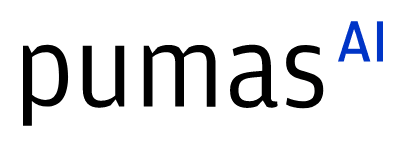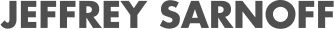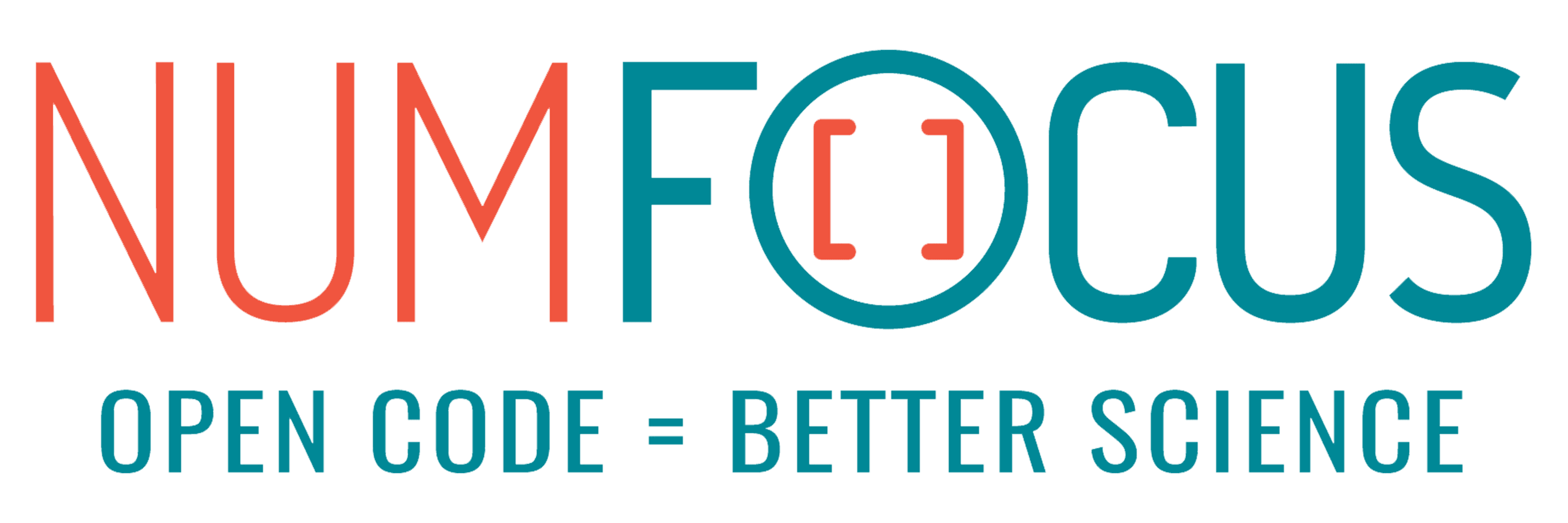# Modia – Modeling Multidomain Engineering Systems with Julia

07/30/2021, 8:10 PM8:20 PM UTC
Green

#### Abstract:

Modia (www.ModiaSim.org) is a set of Julia packages for modeling and simulation of multidomain engineering systems (electrical, 3D mechanical, fluid, etc.). Status and plans of a largely redesigned version of Modia is presented consisting of a new syntax in pure Julia mixing equation with function based modeling (e.g. drive trains + 3D mechanics) and new transformation techniques in order that Modia models can be simulated with the ODE integrators of DifferentialEquations.jl.

#### Description:

Modia (www.ModiaSim.org) is a set of Julia packages for modeling and simulation of coupled multidomain engineering systems (electrical, 3D mechanical, fluid, etc.). It shares many powerful features of the Modelica (www.Modelica.org) language. In the talk status and plans for Modia are presented.

A new simple, yet powerful syntax has been introduced in Modia based on named tuples of Julia and recursive merge. An electrical Resistor can, for example, be defined as:

``````Resistor = OnePort | Model( R = 1.0u"Ω", equation = :[ R*i = v ] )
``````

The | denotes a recursive merge between the named tuple OnePort and a new Model (named tuple) adding a parameter R and Ohms equation, i.e., corresponding to extending the model OnePort having variables and equations. Such a resistor can then be instantiated:

``````R = Resistor | Map(R=0.5u"Ω")
``````

with an updated value of the resistance R. The Model constructor constructs a named tuple which only adds attributes during merge and Map only updates attributes. This use of named tuples unifies and generalizes inheritance, hierarchical modifiers and replaceable models of Modelica. Component instances such as R have ports (defined in OnePort) which are connected to form complete hierarchical system models.

For certain kinds of models, such as multibody systems, the order of evaluating the component equations is independent of the model topology. This means that algorithmic functions can be used for each model component and called according to the connection topology. This avoids repeated structural and symbolic analysis of the multibody equations, the code size is considerably reduced, and pre-compilation is possible. Modia allows to express such kinds of models together with equation-based models.

New symbolic algorithms transform the Modia equations to ODEs (Ordinary Differential Equations in state space form) and generate a Julia function that can be used to simulate the transformed model with ODE integrators of DifferentialEquations.jl.

When instantiating a Modia model, the floating point type of the Modia variables can be defined. This allows for example to easily model uncertainty propagation with Measurements.jl or perform Monte Carlo Simulation with MonteCarloMeasurements.jl. The hierarchical NamedTuple description of a model can be easily mapped to a JSON file. As a result, the complete parameterization of a Modia Model, or the complete Modia model itself, can be exchanged in a straightforward way with a Web App for model composition by drag-and-drop and for 3D animation.

Hilding Elmqvist: Mogram AB Martin Otter, Andrea Neumayr, Gerhard Hippmann: DLR Institute of System Dynamics and Control